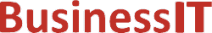O nás     Inzerce     KontaktSpolehlivé informace o IT již od roku 2011[Linux manuál]

# scalb: načte exponent čísla s plovoucí desetinnou čárkou nezávislou na radixech

Originální popis anglicky: scalb - load exponent of a radix-independent floating-point number

Návod, kniha: POSIX Programmer's Manual

## STRUČNĚ

#include <math.h>

double scalb(double x, double n );

## POPIS / INSTRUKCE

The scalb() function shall compute x*r**n, where r is the radix of the machine's floating-point arithmetic. When r is 2, scalb() shall be equivalent to ldexp() . The value of r is FLT_RADIX which is defined in <float.h>.
An application wishing to check for error situations should set errno to zero and call feclearexcept(FE_ALL_EXCEPT) before calling these functions. On return, if errno is non-zero or fetestexcept(FE_INVALID | FE_DIVBYZERO | FE_OVERFLOW | FE_UNDERFLOW) is non-zero, an error has occurred.

## NÁVRATOVÁ HODNOTA

Upon successful completion, the scalb() function shall return x*r**n.
If x or n is NaN, a NaN shall be returned.
If n is zero, x shall be returned.
If x is ±Inf and n is not -Inf, x shall be returned.
If x is ±0 and n is not +Inf, x shall be returned.
If x is ±0 and n is +Inf, a domain error shall occur, and either a NaN (if supported), or an implementation-defined value shall be returned.
If x is ±Inf and n is -Inf, a domain error shall occur, and either a NaN (if supported), or an implementation-defined value shall be returned.
If the result would cause an overflow, a range error shall occur and ±HUGE_VAL (according to the sign of x) shall be returned.
If the correct value would cause underflow, and is representable, a range error may occur and the correct value shall be returned.
If the correct value would cause underflow, and is not representable, a range error may occur, and 0.0 shall be returned.

## CHYBY / ERRORY

The scalb() function shall fail if:
Domain Error
If x is zero and n is +Inf, or x is Inf and n is -Inf.
If the integer expression (math_errhandling & MATH_ERRNO) is non-zero, then errno shall be set to [EDOM]. If the integer expression (math_errhandling & MATH_ERREXCEPT) is non-zero, then the invalid floating-point exception shall be raised.
Range Error
The result would overflow.
If the integer expression (math_errhandling & MATH_ERRNO) is non-zero, then errno shall be set to [ERANGE]. If the integer expression (math_errhandling & MATH_ERREXCEPT) is non-zero, then the overflow floating-point exception shall be raised.

The scalb() function may fail if:
Range Error
The result underflows.
If the integer expression (math_errhandling & MATH_ERRNO) is non-zero, then errno shall be set to [ERANGE]. If the integer expression (math_errhandling & MATH_ERREXCEPT) is non-zero, then the underflow floating-point exception shall be raised.

The following sections are informative.

None.

## APPLICATION USAGE

Applications should use either scalbln(), scalblnf(), or scalblnl() in preference to this function.
IEEE Std 1003.1-2001 only defines the behavior for the scalb() function when the n argument is an integer, a NaN, or Inf. The behavior of other values for the n argument is unspecified.
On error, the expressions (math_errhandling & MATH_ERRNO) and (math_errhandling & MATH_ERREXCEPT) are independent of each other, but at least one of them must be non-zero.

None.

None.

## SOUVISEJÍCÍ

feclearexcept() , fetestexcept() , ilogb() , ldexp() , logb() , scalbln() , the Base Definitions volume of IEEE Std 1003.1-2001, Section 4.18, Treatment of Error Conditions for Mathematical Functions, <float.h>, <math.h> Portions of this text are reprinted and reproduced in electronic form from IEEE Std 1003.1, 2003 Edition, Standard for Information Technology -- Portable Operating System Interface (POSIX), The Open Group Base Specifications Issue 6, Copyright (C) 2001-2003 by the Institute of Electrical and Electronics Engineers, Inc and The Open Group. In the event of any discrepancy between this version and the original IEEE and The Open Group Standard, the original IEEE and The Open Group Standard is the referee document. The original Standard can be obtained online at http://www.opengroup.org/unix/online.html .
 2003 IEEE/The Open Group
©2011-2020 BusinessIT.cz, ISSN 1805-0522 | Názvy použité v textech mohou být ochrannými známkami příslušných vlastníků.
Provozovatel: Bispiral, s.r.o., kontakt: BusinessIT(at)Bispiral.com | Inzerce: Best Online Media, s.r.o., zuzana@online-media.cz
O vydavateli | Pravidla webu BusinessIT.cz a ochrana soukromí | pg(8103)